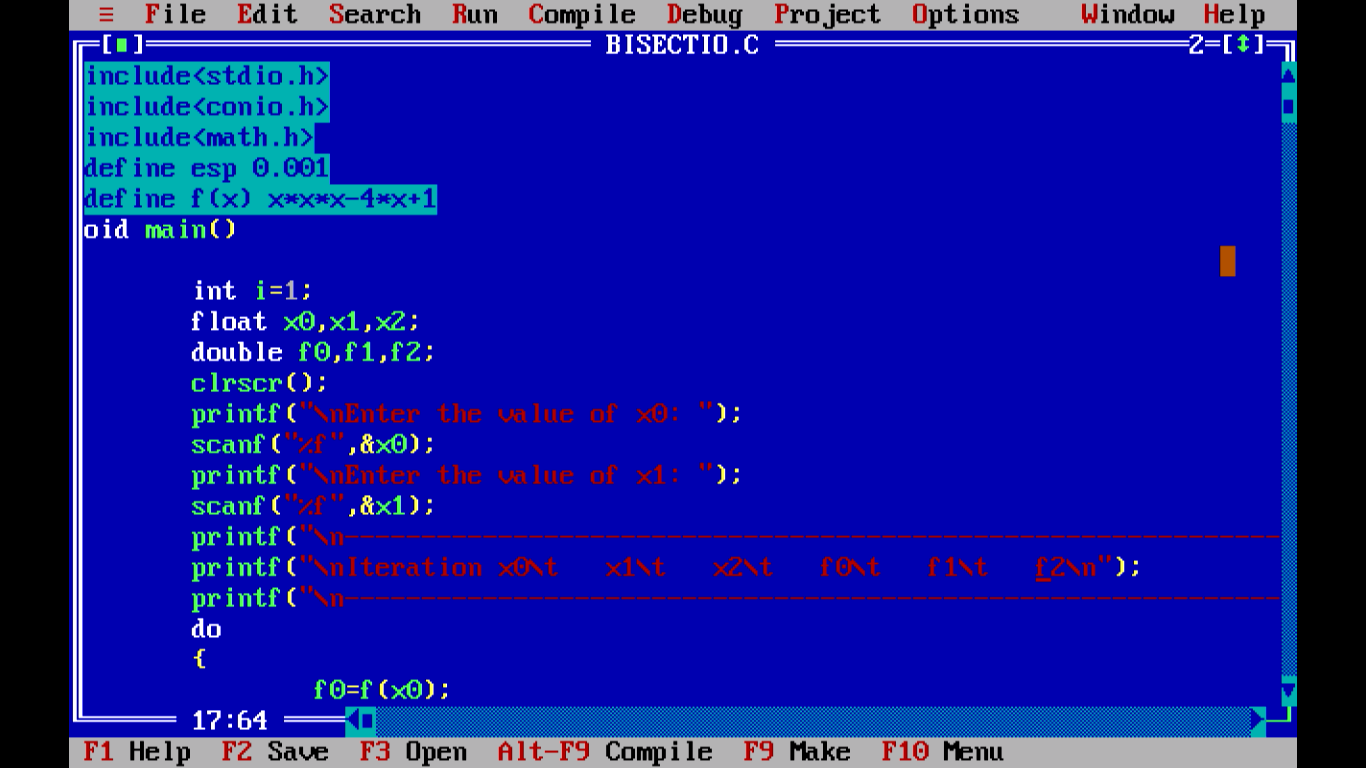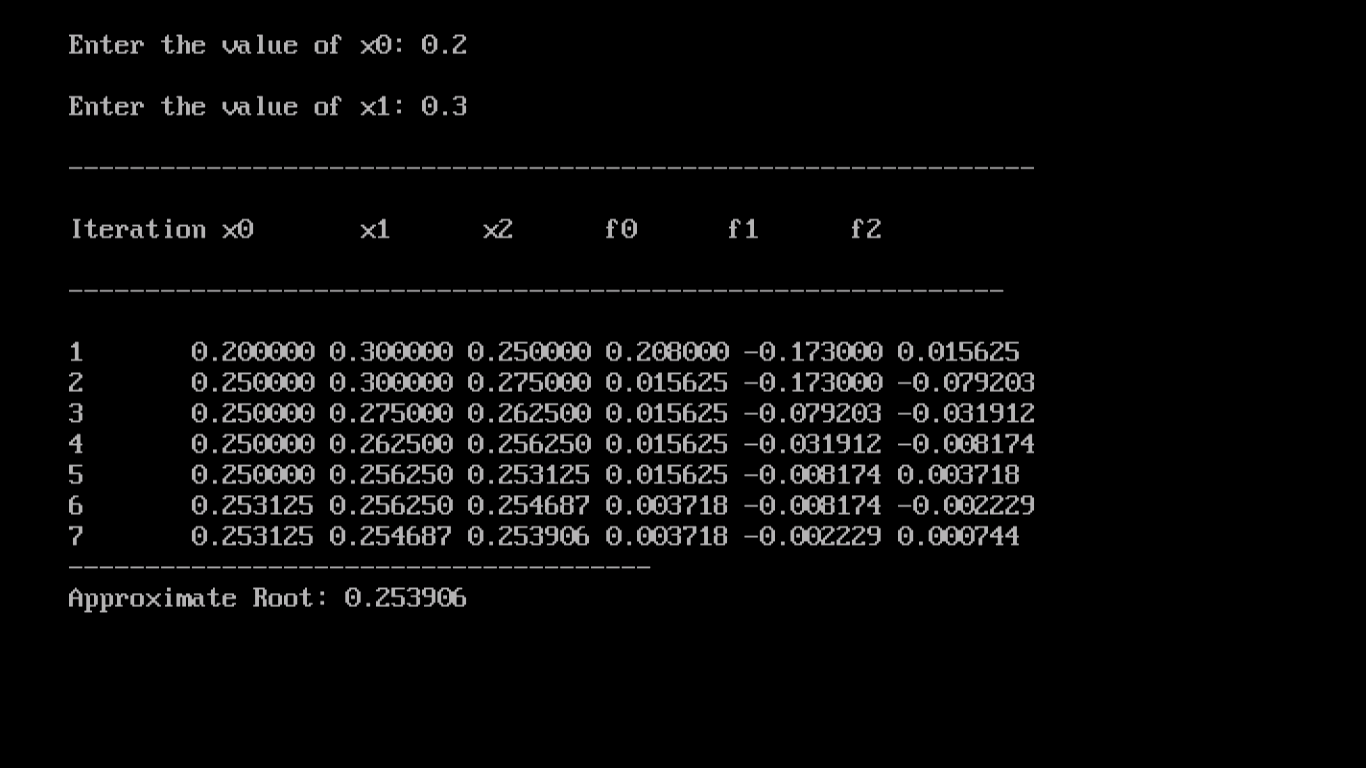# Bisection Method: Algortihm, Flowchart, Program in C

C Programs has multiple application in several branches of science. One such application is in Numerical Analysis. The following article will guide you through the algorithm, flowchart and C Program to evaluate a Bisection Method Numerical Problem using C Language.Bisection Method in C

## What is Bisection Method?

It is an iterative method based on a well known theorem which states that if f(x) be a continuous function in a closed interval [a,b] and f(a)f(b)<0, then there exists at least one real root of the equation f(x)=0, between a and b. If further f'(x) exists and it maintains same sign in [a,b], i.e. f(x) is strictly monotonic, then there is only one real root of f(x)=0, in [a,b]. The method of Bisection is nothing but iterative application of above theorem.

## Computation scheme

1. Find an interval [a0,b0] where f(a0)f(b0)<0 and f'(x) maintains same sign.
2. Write, n(number of iterations), an, bn, xn+1 and f(xn+1) horizontally.
3. Insert +ve or -ve sign with an, as an(+ve) or an(-ve) according as f(a0)>0 or f(a0)<0 and -ve or +ve sign with bn, as bn(-ve) or bn(+ve)  according as f(b0)<0 or f(b0)>0.
4. In (r+1)th iteration, write xr+1 in the column of an(+ve), if f(xr+1)>0 keeping br fixed in the column of bn(-ve). Otherwise, write xr+1 in the column of bn(-ve), of f(xr+1)<0, keeping ar fixed in the column of an(+ve).

## Algorithm

STEP 1: Start
STEP 2: Decide initial values for x1 and x2 and stopping criterion, E.
STEP 3: Compute f1 = f(x1) and f2 = f(x2).
STEP 4: If f1 * f2>0, x1 and x2 do not bracket any root and go to STEP 7;
else continue.
STEP 5: Compute x0 = (x1+x2)/2 and compute f0 = f(x0)
STEP 6: If f1*f0< then
set x2 = x0
else
set x1 = x0
set f1 = f0
STEP 7: If absolute value of (x2 – x1)/x2 is less than error E, then
root = (x1 + x2)/2
write the value of root
go to STEP 7
else
go to STEP 4
STEP 8: Stop.

## FlowchartFlowchart of Bisection Method

## Sample Problem:

WAP in C to find the root of the equation x³ - 4x + 1 = 0 using Bisection Method.

#### Code:

``````#include<stdio.h>                    /*including header files*/
#include<conio.h>
#include<math.h>
#define esp 0.001                    /*defining the error term*/
#define f(x) x*x*x-4*x+1             /*defining the function f(x)*/
void main()
{
int i=1;                     /*declaring variables*/
float x0,x1,x2;
double f0,f1,f2;
clrscr();                    /*clearing screen*/
printf("\nEnter the value of x0: ");
scanf("%f",&x0);      /*generating input/output statements*/
printf("\nEnter the value of x1: ");
scanf("%f",&x1);
printf("\n---------------------------------------------------------------\n");
printf("\nIteration x0\t   x1\t   x2\t   f0\t   f1\t   f2\n");                       /*generating the tabular form*/
printf("\n-------------------------------------------------------------\n");
do                     /*initialization of do loop*/
{
f0=f(x0);
f1=f(x1);
x2=(x0+x1)/2;
f2=f(x2);       /*assigning of values*/
printf("\n%d\t%f %f %f %lf %lf %lf",i,x0,x1,x2,f0,f1,f2);      /*values of the process*/
if(f0*f2<0)     /*the main process*/
x1=x2;
else
x0=x2;
i++;
}while(fabs(f2)>esp);   /*check if the error term is met*/
printf("\n--------------------------------------\n");
printf("Approximate Root: %f",x2); /*final answer*/
getch();
}``````

#### Compiler Window:Compiler Window

#### Output Screen:Output Screen

## Video Tutorial

Feel free to comment below if you are facing problems while executing this program. Visit again soon.